### Example 7.8 Chemical Equilibrium

The following example is used in many test libraries for nonlinear programming and was taken originally from Bracken and McCormick (1968).

The problem is to determine the composition of a mixture of various chemicals satisfying its chemical equilibrium state. The second law of thermodynamics implies that a mixture of chemicals satisfies its chemical equilibrium state (at a constant temperature and pressure) when the free energy of the mixture is reduced to a minimum. Therefore the composition of the chemicals satisfying its chemical equilibrium state can be found by minimizing the function of the free energy of the mixture.

Notation:number of chemical elements in the mixturenumber of compounds in the mixture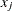number of moles for compound,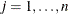total number of moles in the mixture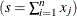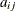number of atoms of elementin a molecule of compound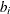atomic weight of elementin the mixture

Constraints for the Mixture:

• The number of moles must be positive: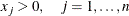• There aremass balance relationships,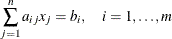Objective Function: Total Free Energy of Mixture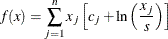with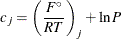where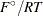is the model standard free energy function for theth compound (found in tables) and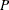is the total pressure in atmospheres.

Minimization Problem:

Determine the parametersthat minimize the objective function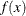subject to the nonnegativity and linear balance constraints.

Numeric Example:

Determine the equilibrium composition of compound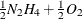at temperature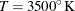and pressure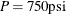.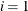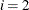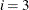Compound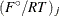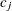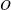1-10.021 -6.089 1 2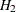-21.096 -17.164 2 3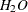-37.986 -34.054 2 1 4-9.846 -5.914 1 5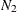-28.653 -24.721 2 6
-18.918 -14.986 1 1 7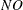-28.032 -24.100 1 1 8-14.640 -10.708 1 9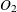-30.594 -26.662 2 10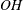-26.111 -22.179 1 1

Example Specification:

proc nlp tech=tr pall;
array c -6.089 -17.164 -34.054  -5.914 -24.721
-14.986 -24.100 -10.708 -26.662 -22.179;
array x x1-x10;
min y;
parms x1-x10 = .1;
bounds 1.e-6 <= x1-x10;
lincon 2. = x1 + 2. * x2 + 2. * x3 + x6 + x10,
1. = x4 + 2. * x5 + x6 + x7,
1. = x3 + x7  + x8 + 2. * x9 + x10;
s = x1 + x2 + x3 + x4 + x5 + x6 + x7 + x8 + x9 + x10;
y = 0.;
do j = 1 to 10;
y = y + x[j] * (c[j] + log(x[j] / s));
end;
run;


Displayed Output:

The iteration history given in Output 7.8.1 does not show any problems.

Output 7.8.1: Iteration History

PROC NLP: Nonlinear Minimization

Trust Region Optimization

Without Parameter Scaling

Iteration   Restarts Function
Calls
Active
Constraints
Objective
Function
Objective
Function
Change
Max Abs
Element
Lambda Trust
Region
1   0 2 3 ' -47.33412 2.2790 6.0765 2.456 1.000
2   0 3 3 ' -47.70043 0.3663 8.5592 0.908 0.418
3   0 4 3   -47.73074 0.0303 6.4942 0 0.359
4   0 5 3   -47.73275 0.00201 4.7606 0 0.118
5   0 6 3   -47.73554 0.00279 3.2125 0 0.0168
6   0 7 3   -47.74223 0.00669 1.9552 110.6 0.00271
7   0 8 3   -47.75048 0.00825 1.1157 102.9 0.00563
8   0 9 3   -47.75876 0.00828 0.4165 3.787 0.0116
9   0 10 3   -47.76101 0.00224 0.0716 0 0.0121
10   0 11 3   -47.76109 0.000083 0.00238 0 0.0111
11   0 12 3   -47.76109 9.609E-8 2.733E-6 0 0.00248

Optimization Results
Iterations 11 Function Calls 13
Hessian Calls 12 Active Constraints 3
Objective Function -47.76109086 Max Abs Gradient Element 1.8637499E-6
Lambda 0 Actual Over Pred Change 0

 GCONV convergence criterion satisfied.

Output 7.8.2 lists the optimal parameters with the gradient.

Output 7.8.2: Optimization Results

PROC NLP: Nonlinear Minimization

Optimization Results
Parameter Estimates
Objective
Function
1 x1 0.040668 -9.785055
2 x2 0.147730 -19.570110
3 x3 0.783153 -34.792170
4 x4 0.001414 -12.968921
5 x5 0.485247 -25.937841
6 x6 0.000693 -22.753976
7 x7 0.027399 -28.190984
8 x8 0.017947 -15.222060
9 x9 0.037314 -30.444120
10 x10 0.096871 -25.007115

Value of Objective Function = -47.76109086

The three equality constraints are satisfied at the solution, as shown in Output 7.8.3.

Output 7.8.3: Linear Constraints at Solution

PROC NLP: Nonlinear Minimization

Linear Constraints Evaluated at Solution
1 ACT -3.608E-16 = 2.0000 - 1.0000 * x1 - 2.0000 * x2 - 2.0000 * x3 - 1.0000 * x6 - 1.0000 * x10
2 ACT 2.2204E-16 = 1.0000 - 1.0000 * x4 - 2.0000 * x5 - 1.0000 * x6 - 1.0000 * x7
3 ACT -1.943E-16 = 1.0000 - 1.0000 * x3 - 1.0000 * x7 - 1.0000 * x8 - 2.0000 * x9 - 1.0000 * x10

The Lagrange multipliers are given in Output 7.8.4.

Output 7.8.4: Lagrange Multipliers

PROC NLP: Nonlinear Minimization

First Order Lagrange Multipliers
Active Constraint Lagrange
Multiplier
Linear EC  9.785055
Linear EC  12.968921
Linear EC  15.222060

The elements of the projected gradient must be small to satisfy a necessary first-order optimality condition. The projected gradient is given in Output 7.8.5.

PROC NLP: Nonlinear Minimization

Free
Dimension
Projected
1 4.5770108E-9
2 6.868355E-10
3 -7.283013E-9
4 -0.000001864
5 -0.000001434
6 -0.000001361
7 -0.000000294

The projected Hessian matrix shown in Output 7.8.6 is positive definite, satisfying the second-order optimality condition.

Output 7.8.6: Projected Hessian Matrix

PROC NLP: Nonlinear Minimization

Projected Hessian Matrix
X1 X2 X3 X4 X5 X6 X7
X1 20.903196985 -0.122067474 2.6480263467 3.3439156526 -1.373829641 -1.491808185 1.1462413516
X2 -0.122067474 565.97299938 106.54631863 -83.7084843 -37.43971036 -36.20703737 -16.635529
X3 2.6480263467 106.54631863 1052.3567179 -115.230587 182.89278895 175.97949593 -57.04158208
X4 3.3439156526 -83.7084843 -115.230587 37.529977667 -4.621642366 -4.574152161 10.306551561
X5 -1.373829641 -37.43971036 182.89278895 -4.621642366 79.326057844 22.960487404 -12.69831637
X6 -1.491808185 -36.20703737 175.97949593 -4.574152161 22.960487404 66.669897023 -8.121228758
X7 1.1462413516 -16.635529 -57.04158208 10.306551561 -12.69831637 -8.121228758 14.690478023

The following PROC NLP call uses a specified analytical gradient and the Hessian matrix is computed by finite-difference approximations based on the analytic gradient:

proc nlp tech=tr fdhessian all;
array c -6.089 -17.164 -34.054  -5.914 -24.721
-14.986 -24.100 -10.708 -26.662 -22.179;
array x x1-x10;
array g g1-g10;
min y;
parms x1-x10 = .1;
bounds 1.e-6 <= x1-x10;
lincon 2. = x1 + 2. * x2 + 2. * x3 + x6 + x10,
1. = x4 + 2. * x5 + x6 + x7,
1. = x3 + x7  + x8 + 2. * x9 + x10;
s = x1 + x2 + x3 + x4 + x5 + x6 + x7 + x8 + x9 + x10;
y = 0.;
do j = 1 to 10;
y = y + x[j] * (c[j] + log(x[j] / s));
g[j] = c[j] + log(x[j] / s);
end;
run;


The results are almost identical to those of the previous run.